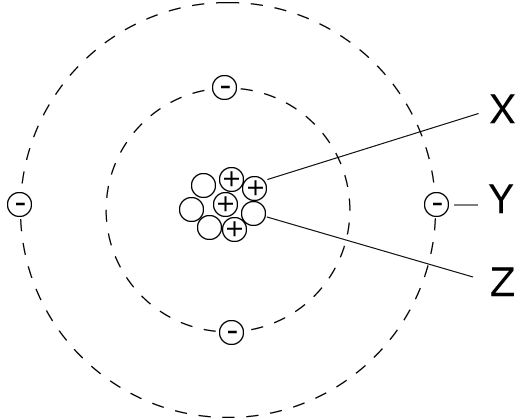Q1-5:

Atoms are made up of three types of particle: proton, neutron and electron.

1. The particle with the smallest mass is the .
2. The particle with a negative charge is the .
3. The particles always present in equal numbers in an atom are …
• A.   protons and neutrons
• B.   protons and electrons
• C.   neutrons and electrons
• D.   protons, neutrons and electrons
4. The particles present in the nucleus of an atom are ….
• A.   protons and neutrons
• B.   protons and electrons
• C.   neutrons and electrons
• D.   protons, neutrons and electrons

5. Isotopes of an element have:

 equal numbers of …. different numbers of… A protons neutrons B protons electrons C neutrons protons D neutrons electrons

6. The diagram of an atom is shown.Particles X, Y and Z represent:

 X Y Z A electron proton neutron B neutron electron proton C proton neutron electron D proton electron neutron

Q7-8:
An atom of magnesium contains 12 protons, 12 electrons and 14 neutrons.

7. What is the electronic configuration of this atom?

• A.  2,8,2
• B.  8,4
• C.  2,8,4
• D.  8,6
8. The mass number of this atom is .
 9. What do the nuclei of 1 H hydrogen atoms contain? 1
• A.   electrons and neutrons
• B.   protons and neutrons
• C.   neutrons only
• D.   protons only

Q10-11:
The table shows information on four particles which are atoms or ions.

 protons neutrons Electronic configuration P 18 22 2,8,8 Q 19 20 2,8,8 R 19 21 2,8,8,1 S 20 20 2,8,8,2
10. Which particles are ions?
• A.   P and Q
• B.   R and S
• C.   Q only
• D.   P, R and S
11. Which particles belong to the same element?
• A.   P and Q
• B.   P and S
• C.   Q and R
• D.   Q and S